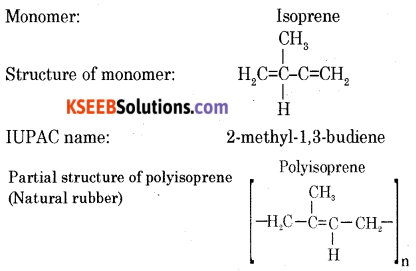# 2nd PUC Chemistry Previous Year Question Paper March 2018

Students can Download 2nd PUC Chemistry Previous Year Question Paper March 2018, Karnataka 2nd PUC Chemistry Model Question Papers with Answers helps you to revise the complete Karnataka State Board Syllabus and score more marks in your examinations.

## Karnataka 2nd PUC Chemistry Previous Year Question Paper March 2018

Time: 3:15 Hours
Max. Marks: 70

Instructions

• The question paper has four parts A, B, C, and D. All parts are compulsory.
• Write balanced chemical equations and draw labeled diagrams wherever required.
• Use Jog tables and simple calculators if necessary (Use of scientific calculators is not allowed).

Part – A

I. Answer all the following questions: (10 × 1 = 10)

Question 1.
State Henry’s law.
At constant temperature, the solubility of a gas in a liquid is directly proportional to the partial pressure of the gas present above the surface of the solution.
p = KH. x
p: partial pressure of the gas.
x: mole fraction of the gas.
KH: Henry’s constant.

Question 2.
Van’t Hoffs factor for a solution is less than one. What is the conclusion drawn from it?

• When the solute undergoes dissociation.
• When the solute undergoes association.
• The solute does not undergo association or dissociation.Question 3.
How many Faraday of electricity is required to reduce 1 mole of MnO4 ions to Mn2+ ions?

Question 4.
If the unit of the rate constant of a reaction is mol-1 LS-1 then mention its order.
Second-order.

Question 5.
Name a metal refined by Van Arkel method.
Zirconium (or) Titanium.

Question 6.
Complete the following equation.
XeF6 + H2O → ____ + 2HF
XeOF4.

Question 7.
What is an ambidentate ligand?
Ligand which can ligate through 2 different atoms is called ambidentate ligand.Question 8.
Name the following reaction.
H3C – Br + AgF → H3C – F + AgBr
Swartz reactionQuestion 9.
Ethanal (CH3CHO) undergoes aldol condensation reaction. Give reason.
Ethanal contains α-hydrogens.

Question 10.
Deficiency of which vitamin causes the disease “Rickets”.
Vitamin – D.

Part – B

Answer any FIVE questions: (Each question carries two marks) (5 × 2 = 10)

Question 11.
What is a Frenkel defect? How does it affect the density of the solid?
It is a type of defect that arises in ionic solids when smaller ion (cation) is dislocated from its normal site to an interstitial site. This defect does not change the density of the solid.

Question 12.
Draw a neat labeled diagram of H2 – O2 fuel cell. Write the reaction that occurs at the cathode of the cell.In this cell, hydrogen and oxygen are bubbled through porous carbon electrodes into concentrated aqueous sodium hydroxide.
Hydrogen is fed into the anode compartment where it is oxidized.
The oxygen is fed into the cathode compartment where it is reduced.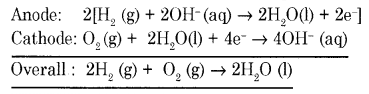Question 13.
A first-order reaction is found to have a rate constant, K = 5.5 × 10-14 S-1. Find the half-life of the reaction.
t½ = 1.26 × 1013 sQuestion 14.
Give reason:
(a) Cerium (Ce) exhibits a +4 oxidation state.
(b) Actinoid contraction is greater from element to element than lanthanoid contraction.
(a) Fe3+ is more stable. Fe3+ has a stable half-filled configuration.
(b) A steady decrease in the size of actinoids with an increase in atomic number is known as actinoid contraction.
Actinoid contraction is greater from element to element in the series due to poor shielding by 5f electrons compared to 4f electrons.

Question 15.
How anisole reacts with bromine in ethanoic acid? Write the chemical equation for the reaction.
Anisole undergoes bromination with bromine in ethanoic acid to form ortho-bromoanisole and para-bromoanisole.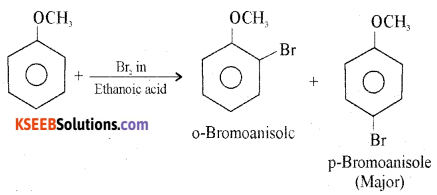Question 16.
Explain the preparation of carboxylic acids from Grignard reagent. Give an equation.
Grignard reagents react with solid carbon dioxide (dry ice) to form a salt of carboxylic acids, which on acidification gives carboxylic acid.Question 17.
Given an example each for
(a) Artificial sweetening agents
(b) Narcotic analgesics.
(a) Chemical compounds which give sweet taste without adding calories.
Ex: Aspartame, Saccharin, Sucralose, Alitame.

(b) Analgesics are chemicals used to reduce body pain without causing impairment
of consciousness.
Ex: Non-norcotic analgesic – Aspirin
Norcotic analgesic – Morphine.Question 18.
What are cationic detergents? Give an example.
Cationic detergents are quaternary ammonium salts of amines with acetates, chlorides or bromides as anions.
Ex: Cetyltrimethylammonium bromideThese are used in hair conditioners.

Part – C

Answer any FIVE of the following: (Each question carries three marks) (5 × 3 = 15)

Question 19.
Explain the process of obtaining “blister copper” from “copper matte” with equations.
Copper matte is a mixture of a cuprous suiphide and little quantity of ferrous Sulphide.
Copper matte along with some quantity of silica is taken in the converter in which hot air is blown.
(a) Remaining ferrous suiphide oxidizes to ferrous oxide, which in turn combines with silica to form slag ferrous silicate
2FeS + 3O2 → 2FeO + 2SO2
FeO + SiO2 → FeSiO3

(b) Part of curious suiphide oxidizes to cuprous oxide which combines with remaining cuprous suiphide forming blister copper.
2Cu2S + 3O2 → 2Cu2O+ 2SO2
2Cu2S + Cu2S → 6Cu + SO2

Question 20.
Write the equations involved in the manufacture of nitric acid by Ostwald’s process by maintaining reaction conditions.
Step 1: Catalytic oxidation of ammonia gives nitric oxide.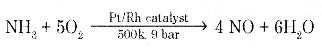Step 2: Nitric oxide thus formed combines with oxygen gives NO2Step 3: Nitrogen dioxide so formed dissolves in water to give HNO3
H2O + 3NO2 → 2HNO3 + NOQuestion 21.
(a) How is ozonized oxygen prepared in the laboratory? Give an equation.
(b) Give the composition of “Oleum”.
When slow dry steam of oxygen is passed through a silent electrical discharge conversion of oxygen to ozone takes place. The product is known as ozonized oxygen.
3O2 → 2O3, ∆H = +142 kJ mol-1

Question 22.
Complete the following equations:
(a) 2NaOH + Cl2 → NaCl + ____ + H2O
(b) Na2SO3 + 2HCl → 2NaCl + H2O + ______
(c) Cl2 + 3F2 $$\underrightarrow { 573K }$$ _____
(a) 2NaOH + Cl2 → NaCl + NaClO + H2O
(b) Na2SO3 + 2HCl → 2NaCl + H2O + SO2
(c) Cl2 + 3F2 $$\underrightarrow { 573K }$$ 2ClF3

Question 23.
How is potassium permanganate (KMnO4) prepared from MnO2? Write the equations.
Potassium perchlorate

Question 24.
(a) Why 3d-series of elements act as good catalysts?
Interstitial compounds are those which are formed by the transitional element when small atoms like H, C, and N are trapped inside the crystal lattices of metals.
Example: VH0.5

(b) Given reason: Ti4+ salts are colorless whereas Cr3+ salts are colored.
Sc3+ ions do not. contain aiìy unpaired electrons hence d-d transition does not take place.
Cr3+ ions contain 3 unpaired electrons, hence d-d transition take place.
Therefore, Cr3+ ions are colored.Question 25.
With the help of the Valence Bond Theory (VBT), explain hybridization, geometry, and magnetic property of [NiCl4]2-.
(i) Orbitals of Ni2+ ion(ii) In Ni2+ ion sp3 hybridization takes place sp3 hybrid orbitals of Ni2+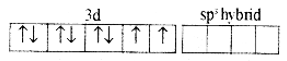(iii) 4 pairs of electrons one from each, occupy the four hybrid orbitals. The complex has tetrahedral geometry and paramagnetic because of two unpaired electrons.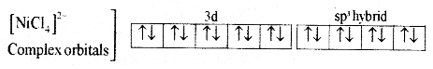For [Ni(Cl)4]2- Complex:

• Type of hybridization: sp3
• Geometry: Tetrahedral
• Magnetic property: Paramagnetic.

Question 26.
(a) Write the IUPAC name of [Co(NH3)4(H2O)Cl]Cl2.
Tetraammineaquachloridocobalt(III) chloride

(b) Explain linkage isomerism with example.
Two or more co-ordination compounds have the same molecular formula but differ in the mode of attachment of the ambident ligand to the central metal ion.
Ex: [Co(NH3)5(NO2)]Cl2
Pentaamminenitrito-N-cobalt(III) chloride
Ex: [Co(NH3)5(ONO)]Cl2
Pentaamminenitrito-O-cobalt(III) chloride

Part – D

Answer any three of the following: (Each question carries five marks) (3 × 5 = 15)

Question 27.
(a) Calculate packing efficiency in a simple cubic lattice.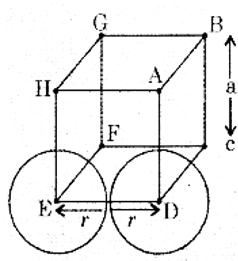In simple cubic lattice, the atoms are located only on the corners of the cube.
The atoms touch each other along the edge.
The edge length of the cube is ‘a’. and radius or each atom is r.
a = 2r
The volume of the unit cell is = a3 = (2r)3 = 8r3
Simple cubic mut cells contain only one atom.
The volume of the one atom = $$\frac{4}{3} \pi r^{3}$$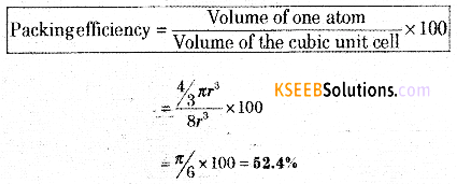(b) An element having an atomic mass 107.9 u has FCC lattice. The edge length of its unit cell is 408.6 pm. Calculate the density of the unit cell. [Given, NA = 6.022 × 1023 mol-1].
6.242 g cm-3Question 28.
(a) The boiling point of benzene is 353.23K. When 1.80 g of a non-volatile, non-ionizable solute was dissolved in 90g of benzene, the boiling point raised to 354.11 K. Calculate the molar mass of the solute. [Kb for benzene = 2.53K Kg mol-1].
The boiling point of solution (T) = 354.11 K.
The boiling point of the solvent (T°) = 353.23 K.
Elevation in boiling point. (∆Tb) = T – T° = 354.11 – 353.23 = 0.88 K.
Mass of solute = W2 = 1.80 g
Mass of solvent = W1 = 90 g
Kb = 2.53 K.kg mol-1
Molar mass of solute = M2 = ?(b) Define:

1. Molality of a solution.
2. Isotonic solutions.

1. Molality is defined as the number of the solute present in one kilogram of solvent.
2. Two solutions having the same osmotic pressure at a given temperature are called isotonic solutions.
Example: Blood cells are isotonic with 0.9 (m/v) sodium chloride solution (saline).

Question 29.
(a) Calculate e.m.f of the cell for the reaction
Mg(s) + Cu2+ (0.0001 M) → Mg2+ (0.001 M) + Cu(S)
Given that: $$\mathbf{E}_{\mathrm{Mg}^{2+}}^{0}$$ = -2.37 V, $$\mathbf{E}_{\mathrm{Cu}^{2+} / \mathrm{C}}^{\mathbf{0}}$$ = +0.34V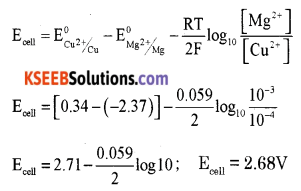(b) (i) State Kohlrausch law.
Limiting the molar conductivity of an electrolyte can be represented as the sum of the individual contributions of the anion and cation of the electrolyte.
If the molar conductivity of cation is denoted by $$\lambda_{+}^{0}$$ and that of anion $$\lambda_{-}^{0}$$
$$\wedge_{m}^{\circ}=v_{+} \lambda_{+}^{0}+v_{-} \lambda_{-}^{0}$$
Where v+ and v are the number of cations and anions per formula unit of an electrolyte.

(ii) What is meant by limiting molar conductance?
Molar conductivity of an electrolyte solution at infinite dilution (when concentration approaches zero) is called limiting molar conductivity.Question 30.
(a) Derive an integrated rate for the first-order reaction.
Consider a zero-order reaction.
R → P
$$\frac{-d[R]}{d t}$$ = k[R]1
where k is velocity constant of n first order reaction.
$$\frac{-d[R]}{d t}$$ = -k.dt
Integrating the equation
∫$$\frac{-d[R]}{d t}$$ = -∫k.dt
ln[R] = -kt + I ……. (1)
I is constant of integration
When t = 0, [R] = [R]0 where [R]0 is initial concentration of the reactant.
ln[R]0 = -k × 0 + 1
I = ln[R]0
Substituting I value in equation (1)
ln[R] = -kt + ln[R]0
kt = ln[R]0 – ln[R]
$$k=\frac{2.303}{t} \log \frac{[R]_{0}}{[R]}$$

(b) Draw a graph of potential energy V/S reaction co-ordinates showing the effect of catalyst on activation energy (Ea) of a reaction.Catalyst provides an alternate pathway by reducing the activation energy and lowering the energy barrier. More molecules enter the threshold energy state leading to chemical reaction and hence increases the rate.

Question 31.
(a) Write any two differences between lyophilic and lyophobic colloids.
Lyophilic sols (Colloids)

• Colloidal particles have an affinity for dispersion medium.
• These are very stable.
• These are reversible in nature.
• Viscosity is higher than that of the dispersion medium.

Lyophobic sols (Colloids)

• Colloidal particles do not have an affinity for dispersion medium.
• These are unstable.
• These are irreversible in nature.
• Viscosity is the same as that of the dispersion medium.(b) What is heterogeneous catalysis? Give an example.
A catalytic process in which the reactants and the catalyst are in different phases is known as heterogeneous catalysis.
Example (1): Oxidation of sulphur dioxide to sulphur trioxide in presence of platinum catalyst.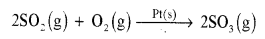Example (2): Combination of hydrogen and nitrogen to form ammonia.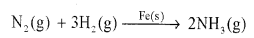Example (3): Hydrogenation of vegetable oil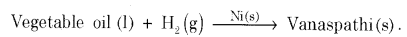(c) Give an expression for Freundlich adsorption isotherm.
$$\frac{x}{\mathrm{m}}=\mathrm{K} \cdot p^{1 / n}$$
x is mass of the gas adsorbed.
m is mass of the adsorbent.
p is pressure.
K1 and n are constants.

Answer any four of the following. Each question carries five marks: (4 × 5 = 20)

Question 32.
(a) Write the equations for the steps in the SN1 mechanism of the conversion of tert-butyl bromide into tert-butyl alcohol.
The reaction between tert-butyl bromide and hydroxide ion gives tert-butyl alcohol. The rate of reaction depends only on the concentration of tert-butyl bromide. Hence it is a nucleophilic first order substitution reaction.Mechanism:
Step 1: Polarised C — Br undergoes cleavage to produce planar carbocation.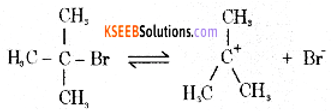Step 2: The carbocation is then attacked by a nucleophile (OH) on either side to form tert-butyl alcohol.(b) Explain the Fitting reaction.
when aryl halide treated with sodium in ether medium two aryl groups join together to form biphenyl.(c) Name the reagent used in the dehydrohalogenation of haloalkanes.
Alcoholic KOH.Question 33.
(a) Write the mechanism of acid-catalyzed dehydration of ethanol to ethene.
Step 1: Formation of protonated alcohol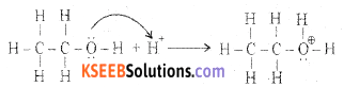Step 2: Formation of carbocationStep 3: Formation of ethene by the elimination of a proton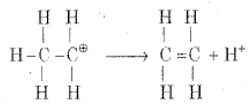(b) Between phenol and alcohol which is more acidic? Why?
Phenols are more acidic than alcohols. This is because the phenoxide ion formed from the ionization of phenol is stabilized by resonance.

Question 34.
(a) Explain Rosenmund reduction with the equation.
When benzoyl chloride (acid chloride) is hydrogenated with catalyst palladium on barium sulphate, benzaldehyde is obtained.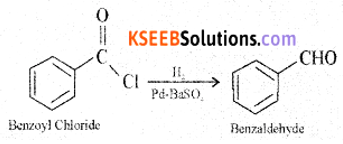(b) How does propanone (CH3COCH3) react with hydrazine? Give an equation.
Propanone undergoes a nucleophilic addition reaction with hydrazine forming addition product Hydrazone.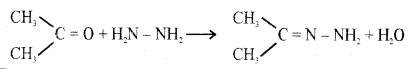(c) Name an oxidising agent used in the Etard’s reaction.
Chromyl Chloride (CrO2Cl2)

Question 35.
(a) Explain carbyl amine reaction with the equation.
Primary amines on heating with chloroform and alcoholic KOH form carbylamines.(b) How does nitrobenzene is reduced to aniline? Give an equation.
Nitro compounds are reduced to amines by pacing hydrogen ga in the presence of finely divided nickel, palladium, or platinum catalyst.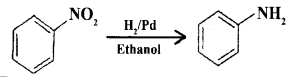(c) Write the IUPAC name of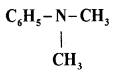N, N – DimethylbenzanamineQuestion 36.
(a) Write the Haworth structure of “Lactose”.(b) (i) What are non-essential amino acids?
Amino acids that are synthesized in the body are known as non-essential amino acids.
Example: Glycine, Alanine.
Amino acids that are not synthesized in the body and must be supplied through the diet are known as essential amino acids.
Example: Lysine, Histidine.

(ii) Write the Zwitterionic structure of “glycine”.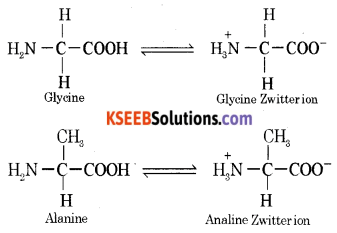(c) Name the nitrogenous base present in RNA but not in DNA.
Uracil

Question 37.
(a) Explain the preparation of Nylon-6, 6 with the equation.
It is obtained by the condensation polymerization of hexamethylene diamine with adipic acid under high pressure and at high temperatures.(b) What are thermoplastic polymers? Give an example.